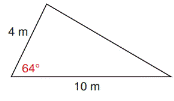Chapter 11.4, Problem 14EElementary Geometry For College St...

7th Edition
Alexander + 2 others
ISBN: 9781337614085

Solutions

Chapter
SectionElementary Geometry For College St...

7th Edition
Alexander + 2 others
ISBN: 9781337614085
Textbook Problem

In Exercises 11 to 14, find the area of each triangle shown. Give the answer to the nearest tenth of a square unit.To determine

To find:

The area of the given triangle

Explanation

Formula:

The area of the triangle is given by,

A=12absinγ unit2.,

A=12bcsinα unit2.,

A=12acsinβ unit2.,

Where a, b, and c are the measures of sides of the triangle, α, β, and γ are the acute angle of the triangle.

Calculation:

Given,

Still sussing out bartleby?

Check out a sample textbook solution.

See a sample solution

The Solution to Your Study Problems

Bartleby provides explanations to thousands of textbook problems written by our experts, many with advanced degrees!

Get Started